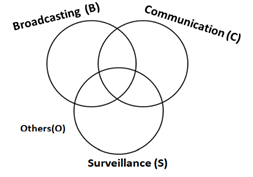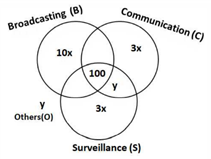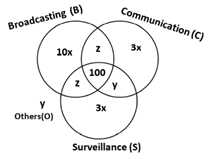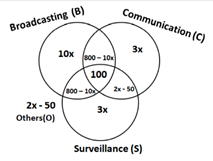#### Tables and Caselets

Logical Reasoning and Data Interpretation (LRDI) in CAT or any other non-CAT exam, is about analyzing and interpreting data and bringing it to a meaningful conclusion. The huge pile of data needs to be sanitized, categorized, and then simplified into some information that can help in decision making. As a future manager, you need to understand how to translate this data into relevant information and figures.

LRDI in CAT, XAT, and other exams have questions on Tables and Caselets every year. Data is given in the form of statements in Caselets, which is then converted into a table and then solved.

Importance of Tables and Caselets in various exams

CAT – In the LRDI section, most of the questions are in the form of caselets.  A single set of caselet may have 3-4 questions. Few direct sets on Tables may be present. The sets are designed such that 1 to 2 questions can be attempted from each set.
 Year No of Sets No of Questions Good attempt Difficulty 2019 Slot 1 4 16 12 Moderate Slot 2 3 12 10 Moderate to Difficult 2018 Slot 1 5 19 15 Easy to Moderate Slot 2 5 20 16 Moderate

XAT – Questions on Tables and Caselets in XAT mostly range from 2 to 3; the question may appear complex, but you need to read the question carefully and note the details mentioned. Every set has at least one question that is easy and can be attempted.

 Year No of Sets No of Questions Good attempt Difficulty 2020 3 10 6 Difficult 2019 2 6 4 Moderate

SNAP – Questions on Tables and Caselets in SNAP range from 1 to 2.  SNAP follows a slightly different pattern. Small caselets of a single question each may also be present.

 Year No of Sets No of Questions Good attempt Difficulty 2019 2 8 5 Moderate 2018 2 8 6 Moderate

MAT – The pattern and difficulty level of MAT is different from all the above-mentioned exams. There are no Caselet type questions. Data is usually given in the table itself. All the questions are pretty much straightforward.

 Year No of Sets No of Questions Good attempt Difficulty 2020 1 5 4 Easy 2019 1 5 4-5 Easy

### List of Concepts in that Chapter

There are two types in which Caselets are prepared. Caselets and Tables questions in CAT, XAT, SNAP, etc. are of the following types:

1. Reasoning based paragraph – Caselets of this type tests the application of various Reasoning concepts. Few types of caselet within this category are:

1.1. Sitting Arrangement – In this type, few people or things are arranged, and information is given in the form of relative positioning. You are supposed to decode their positions and answer the questions based on that

1.2. Games and Tournaments – Caselet with the information of different players, rules of the game, and the number of matches played or to be played are given. You must interpret this information and answer the questions that follow

2. Numerical Data based paragraph – This type of Caselet usually comes under the Data Interpretation part of different exams. There are no set concept or type for such caselets, it can be any data from which information needs to be extracted. The next step is to form the Table and solve the question

### Caselets and Tables – previous year questions

Question 1 – A supermarket has to place 12 items (coded A to L) on shelves numbered 1 to 16. Five of these items are types of biscuits, three are types of candies and the rest are types of savories. Only one item can be kept on a shelf. Items are to be placed such that all items of the same type are clustered together with no empty shelf between items of the same type and at least one empty shelf between two different types of items. At most two empty shelves can have consecutive numbers.

The following additional facts are known:

1. A and B are to be placed on consecutively numbered shelves in increasing order
2.  I and J are to be placed in consecutively numbered shelves both higher numbered than the shelves in which A and B are kept
3. D, E, and F are savories and are to be placed on consecutively numbered shelves in increasing order after all the biscuits and candies
4.  K is to be placed on shelf number 16
5. L and J are items of the same type, while H is an item of a different type
6. C is a candy and is to be placed on a shelf preceded by two empty shelves
7. L is to be placed on a shelf preceded by exactly one empty shelf  [CAT – 2019/ Slot-I]

Total number of Biscuits = 5

Total number of Candies = 3

So, Total number of Savouries = 12 – 5 – 3 = 4

From point iii) and iv) it is clear that D, E, F and K are 4 savouries and are kept in shelves numbered 13,14, 15 and 16 as there is no empty shelf between items of the same type.

From point II), V and VII) I, J and L are of the same type L being in the least numbered shelf among 3.

As from point VI,) C is candy so I, J and L must be Biscuits as there are only 3 candies.

From point V) H is not Biscuits so it must be a candy thus A and B must be Biscuits. Now Item can be placed as given below.

Case 1) when all biscuits are placed after candies.

Case 2) When all candies are placed after biscuits.

 1 2 3 4 5 6 7 8 9 10 11 12 13 14 15 16 Case 1 C H/G G/H L A B I/J J/I D E F K Case 2 L A B I/j J/I C H/G G/H D E F K
Total 8 cases are possible. All questions can be answered now.
1. There are two empty shelves between the biscuits and the candies.
2. All candies are kept before biscuits.
3. All biscuits are kept before candies.
4. There are at least four shelves between items B and C

Question 2: Six players – Tanzi, Umeza, Wangdu, Xyla, Yonita, and Zeneca competed in an archery tournament. The tournament had three compulsory rounds, Rounds 1 to 3. In each round, every player shot an arrow at a target. Hitting the center of the target (called bull’s eye) fetched the highest score of 5. The only other possible scores that a player could achieve were 4, 3, 2, and 1. Every bull’s eye score in the first three rounds gave a player one additional chance to shoot in the bonus rounds, Rounds 4 to 6. The possible scores in Rounds 4 to 6 were identical to the first three.

A player’s total score in the tournament was the sum of his/her scores in all rounds played by him/her. The table below presents partial information on points scored by the players after completion of the tournament. In the table, NP means that the player did not participate in that round, while a hyphen means that the player participated in that round and the score information is missing:

 Round-1 Round-2 Round-3 Round-4 Round-5 Round-6 Tanzi – 4 – 5 NP NP Umeza – – – 1 2 NP Wangdu – 4 – NP NP NP Xyla 5 5 5 1 5 – Yonita – – 3 5 NP NP Zeneca – – – 5 5 NP
The following facts are also known:
1. Tanzi, Umeza, and Yonita had the same total score
2. Total scores for all players, except one, were in multiples of three
3. The highest total score was one more than double of the lowest total score
4. The number of players hitting bull’s eye in Round 2 was double that in Round 3
5. Tanzi and Zeneca had the same score in Round 1 but different scores in Round 3  [CAT-2019/ Slot-II]

As from the table given we can see that Xyla had played all the six rounds in  he had scored 5 points in each of first 3 rounds. Similarly Tanzi and Yonita each had hit one bull’s eye score in first three rounds, Umeza and Zeneca had two bull’s eye score in first 3 rounds while Wangdu didn’t had any bull’s eye score. So this information can be tabulated as below: (Name of players have been represented by first letter of their name )

 Round-1 Round-2 Round-3 Round-4 Round-5 Round-6 Total T a 4 c 5 NP NP x U – – – 1 2 NP x W – 4 – NP NP NP X 5 5 5 1 5 – Y – – 3 5 NP NP x Z a – d 5 5 NP
As number of player hitting bull’s eye in round 2 is double that of the number in round 3 and 2 players have already scored 4 points in Round 2 so maximum possible people hitting bulls eye in Round 3 can be 2 and in round 2 can be 4.
Thus a = 5 as c and d can not be 5.
Possible score of U = 5+5+1+2 + b = 13+b as it should be multiple of 3 so b = 2 thus x = 15
Thus Tanzi’s score in round 3 , c = 15 – 5 – 4 – a = 15 – 5 -4-5 = 1
Also W must have minimum score as his maximum possible score = 12 (if he scores 4 in each of first 3 rounds)Let it be m and X must have maximum possible score let it be n.
As m <= 12 and n >= 22
As n= 2m + 1
So, n can be 23 or 25
But if n = 23 , m = 11 (which is not possible as only one of the all total score is not multiple of 3)
Thus n = 25 and m = 12
As Z has four scores of 5 so his 5th score will be either 1 or 4. But it can not be 1 as T’s score was 1.
Now the complete table will look like,
 Round-1 Round-2 Round-3 Round-4 Round-5 Round-6 Total T 5 4 1 5 NP NP 15 U 2 5 5 1 2 NP 15 W 4 4 4 NP NP NP 12 X 5 5 5 1 5 4 25 Y 2 5 3 5 NP NP 15 Z 5 5 4 5 5 NP 24

Question 3 – 600 satellites were sent up by a country for several purposes. The purposes are classified as broadcasting (B), communication (C), surveillance (S), and others (O). A satellite can serve multiple purposes, however, a satellite serving either B, or C, or S does not serve O. The following facts are known about the satellites:

1. The numbers of satellites serving B, C, and S (though maybe not exclusively) are in the ratio 2:1:1

2. The number of satellites serving all three of B, C, and S is 100

3. The number of satellites exclusively serving C is the same as the number of satellites exclusively serving S. This number is 30% of the number of satellites exclusively serving B

4. The number of satellites serving O is the same as the number of satellites serving both C and S but not B

Q1) What best can be said about the number of satellites serving C? [CAT-2018/ Slot-II]

It is given that a satellite serving either B, or C, or S does not serve O. So we can say that it’s basically 3 satellites broadcasting (B), communication (C), surveillance (S) which can have intersections. Those satellites which are not part of any category are placed in others. We can draw the Venn diagram as follows:1. The numbers of satellites serving B, C, and S (though may be not exclusively) are in the ratio 2:1:1.
2. The number of satellites serving all three of B, C, and S is 100.
3. The number of satellites exclusively serving C is the same as the number of satellites exclusively serving S. This number is 30% of the number of satellites exclusively serving B.
4. The number of satellites serving O is the same as the number of satellites serving both C and S but not B.
Let ’10x’ be the number of satellites exclusively serving B. Then, the number of satellites exclusively serving C and S = 0.30*10x = 3xLet ‘y’ be the number of satellites serving others(O).
Let ‘z’ be the number of satellites serving B, C but not S. Since the numbers of satellites serving B, C, and S (though may be not exclusively) are in the ratio 2:1:1. Therefore, we can can say that number of satellites serving B, S but not C = z.It is given that:
10x+2z+2y+6x = 1600 ⇒ 8x+z+y = 750 … (1)
The numbers of satellites serving B, C, and S (though may be not exclusively) are in the ratio 2:1:1.
10x + 2z + 100 = 2(z + 100 + 3x + y) ⇒ 2x = 50 + y ⇒ y = 2x – 50 … (2)
We can substitute this in equation (1) 8x+z+2x – 50 = 750 ⇒  z = 800 – 10x … (3)Let us define boundary condition for x, 2x – 50 >= 0 ⇒  x >= 25
Also, 800 – 10x >= 0 ⇒ x <= 80
Therefore, we can say that x ϵ [25, 80]. The number of satellites serving C = 800 – 10x + 100 + 3x + 2x – 50 = 850 – 5x
At x = 25, The number of satellites serving C = 850 – 5x = 850 – 5*25 = 725
At x = 80, The number of satellites serving C = 850 – 5x = 850 – 5*80 = 450
Hence, we can say that the number of satellites serving C must be between 450 and 725. Hence, option C is the correct answer.

### How to Prepare

Preparing any topic from the scratch requires patience, hard work, and above all commitment. Tables and Caselets, not only require a thorough knowledge of all the concepts, but they also require a few tricks and shortcuts to be followed. Here are some Level-wise preparatory guidelines to follow:

Level – 1

1. Know your basics. All the types of Logical Reasoning questions should be tried before coming to Caselets and Tables in Reasoning based Caselet
2. Learn Speed calculation: For an effective and quick calculation, be thorough with tables till 20, memorize squares till 30, and cubes till 15. Extracting data from caselets into a table consumes most of the time; speedy calculation tricks can help you analyze data in the table quickly
3. Fraction – Percentage relation: Most of the questions require approximate calculations, knowing the percentage – fraction conversion will help solve the problem quickly
4. Practice beginner level questions on Arrangements, Games and Tournaments, and numerical caselets

Level – 2

1. Move on to more complex problems, attempt beginner and intermediate level mock on Tables and Caselets
2. Solve previous year questions on Tables and Caselets and keep a watch on time. Be careful while going through each point, a slight mistake in analyzing a single point will lead to a completely wrong solution
3. Keep attempting mocks to check your performance
4. Topic-wise mocks, provided by MBAP, can be utilized to enhance your performance

Level – 3

1. For advanced level preparation, start practising questions from “How to prepare for Data Interpretation” and “How to prepare for Logical Reasoning” by Arun Sharma
2. Questions in the books are categorized into Level of Difficulty (LOD); based upon your preparation level, start attempting 3 or 4 questions daily